nextnano.com  GUI: nextnanomat  Tool: nextnano++  Tool: nextnano³  Tool: nextnano.QCL  Download | Search | Copyright | News | Publications  * password protected nextnano³ software1D QW (NEGF)

# nextnano3 - Tutorial

## Applying the NEGF method to a quantum well

Author: Stefan Birner, Tillmann Kubis

If you are interested in the input files that are used within this tutorial, please contact stefan.birner@nextnano.de.
`-> 1DNEGF_InGaAs_QW_ballistic_CBR.in    - `nextnano3 input file using the CBR method``` -> 1DNEGF_InGaAs_QW_ballistic.in        - ``` nextnano3 input file using the NEGF method``` -> 1DNEGF_InGaAs_QW_scattering.in       - ``` nextnano3 input file using the NEGF method``` -> 1DNEGF_InGaAs_QW_scattering_bias.in  - ```nextnano3 input file using the NEGF method```  ```

## Applying the NEGF method to a quantum well

This tutorial is based on the following paper:

`[Kubis_HCIS14_2005]`
Self-consistent quantum transport theory of carrier capture in heterostructures
T. Kubis, A. Trellakis, P. Vogl
Proceedings of the 14th International Conference on Nonequilibrium Carrier Dynamics in Semiconductors, M. Saraniti and U. Ravaioli, eds., Chicago, USA, July 25-19, 2005, Springer Proceedings in Physics, vol. 110, pp. 369-372

### Quantum well structure - InGaAs/GaAs n-i-n heterostructure

• 12 nm In0.14Ga0.86As QW in the center surrounded on each side by GaAs barriers (of width 19 nm each)
```z-coordinates = 19.0d0  31.0d0   ! 19 - 31 [nm]```
• Doping: n-i-n
GaAs n-i-n structure with an InGaAs quantum well within the intrinsic region.```  -  0 nm - 17 nm: ```17 nm n-doped region with n = 1 * 1018 cm-3```  - 17 nm - 33 nm: ```16 nm intrinsic region, i.e. 12 nm InGaAs QW + 2 nm in GaAs barriers on each side```  - 33 nm - 50 nm: ```17 nm n-doped region with n = 1 * 1018 cm-3
• T = 300 K (room temperature)
• device length = 50 nm
``` z-coordinates = 0.0d0  50.0d0    ! 0 - 50 [nm]```
• constant effective electron mass throughout the device (parabolic dispersion): m* = 0.067 m0 (GaAs)
``` conduction-band-masses = 0.067d0  0.067d0  0.067d0 ! [m0] ```electron mass of GaAs``` ```Note: This NEGF code is so general that it can take into account a spatially varying, material dependent, nonparabolic effective electron mass.
To keep this tutorial as simple as possible, we use here the GaAs mass also for the InGaAs QW.
• Dielectric constants
``` static-dielectric-constants  = 12.93d0 12.93d0 12.93d0 ! ```epsilon(0)```  optical-dielectric-constants = 10.89d0                 ! ```epsilon(infinity)``` ```Note: This NEGF code is so general that it can take into account spatially varying, material dependent dielectric constants.
To keep this tutorial as simple as possible, we use here the GaAs values also for the InGaAs QW.
• constant polar optical phonon energy throughout the device: 0.035 eV (GaAs)
` LO-phonon-energy = 0.035d0 ! [eV]`
• GaAs/In0.14Ga0.86As conduction band offset: CBO = 0.150 eV
(For simplicity, we do not take into account strain.)
• Grid resolution: 1 nm, i.e. 49 grid points in total (plus the two boundary points).
This corresponds to 50 equidistant grid points within the NEGF part.
• Ohmic contacts: The two reservoirs at the left and right boundaries are in thermal equilibrium.

## Ballistic NEGF calculation

`-> 1DNEGF_InGaAs_QW_ballistic.in`

• VSD = 0 eV       (source-drain), see Fig. 1 (a) in` [Kubis_HCIS14_2005]`
• ballistic device (absence of any scattering)
• electron-electron scattering in Hartree approximation, see Fig. 1 (a) in``` [Kubis_HCIS14_2005]```

### Necessary feature for ballistic calculation

``` \$scattering-mechanisms   ...   ballistic             = yes      ! ```Fig. 1(a): switch off scattering (ballistic calculation) - to make calculation faster

### Doping profile

The following figure shows the doping profile of the structure.
The structure is n-type doped (n = 1 * 1018 cm-3) with a 16 nm intrinsic region in the center.
The conduction band edge is also indicated to indicate the position of the 12 nm wide InGaAs quantum well.
The output for the doping profile can be found in the following files:
`- doping_concentration1D.dat - `doping profile as specified in input file for each doping function``` - doping_profile1D.dat       - ```total doping profile as specified in input file (n-type + p-type)``` - NEGF/dopingV_new.dat       - ```doping profile used by NEGF code (should by n-type)### Conduction band edge

The following figure shows the conduction band edge energy of the GaAs/InGaAs heterostructure.
The black line is the band edge that is input for the calculation. It can be found in this file:
`  NEGF/ex_potential_new.dat  `(in units of [eV])
The red line is the self-consistently calculated conduction band profile (calculated by NEGF method) and can be found in this file:
`  NEGF/pot_new.dat           `(in units of [eV])

Note: The conduction band edge of nextnano³ is usually contained in this file:
`  band_structure/cb1D_001.dat        `(in units of [eV]) (or)### Energy-resolved electron density

The following figure on the left shows the contour plot of the local, energy-resolved electron density n as a function of position z and energy E at zero bias and in the absence of any scattering (ballistic device) for the n-i-n GaAs structure with an InGaAs quantum well in the center.
n(z,E) is in units of [1018 eV-1cm-3].
Integrating n(z,E) over the energy E yields the electron density n(z).
The black line shows the self-consistently calculated conduction band edge profile.
The figure on the right shows a contour plot of the energy-resolved local density of states LDOS(z,E) in units of [eV-1 Angstrom-1].
The LDOS(z,E) is known as the spectral function.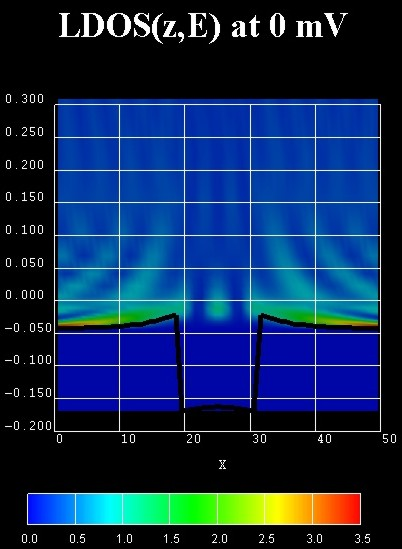The zero of energy is put at the Fermi level of the left contact.
In the calculation, the Hartree Coulomb interaction was taken into account (Poisson equation).

Due to the absence of inelastic scattering, the carriers cannot fill the quantum well but occupy a resonance that is derived from the third quantum well state. This explains the interference pattern in the well region at room temperature.

The energy-resolved electron density n(z,E) can be found in this file:
`   NEGF/density_energy_resolved.fld - `in units of [1018 cm-3 eV-1]      (AVS format)``` ```The energy-resolved electron density n(z,Ez) can be found in this file:
`   NEGF/density_Ez_energy_resolved.fld - `in units of [1018 cm-3 eV-1]      (AVS format)``` ```The energy-resolved local density of states (spectral function) LDOS(z,E) can be found in this file:
```   NEGF/spectral_real_avs.fld         - ```in units of [eV-1 Angstrom-1]   (AVS format)``` ```The conduction band profile can be found in this file:
```   NEGF/pot_avs.fld                   - ```in units of [eV]```           ``` (AVS format)
```   NEGF/pot_new.dat                   - ```in units of [eV]

## NEGF calculation including scattering - zero bias (equilibrium)

`-> 1DNEGF_InGaAs_QW_scattering.in`

Acoustic (both, elastic and inelastic) and polar-optical phonon scattering, electron-electron scattering in Hartree approximation, see Fig. 1 (b) and 2 (a) in` [Kubis_HCIS14_2005]`.

• VSD = 0 eV       (source-drain), see Fig. 1 (b) in` [Kubis_HCIS14_2005]`.

The following scattering mechanisms were used:

``` \$scattering-mechanisms   !----------------------------------------------------------------------   ! ```Note:` lattice_constant a `and``` sound_velocity v ```determine the```   ! ```dispersion relation of acoustic phonons:``` E_LA = h_bar v q ```where` q `is from` 0 `to` pi/a`.```   !----------------------------------------------------------------------   acoustic_phonons      = inelastic ! ```inelastic acoustic phonon scattering``` ! acoustic_phonons      =   elastic ! ```   elastic acoustic phonon scattering - as in paper (Fig. 1(b))```   lattice_constant      = 5.6534d0 ! ```GaAs` [Angstrom] - `Note:``` [Angstrom] ```not``` [nm].   sound_velocity        = 5.2d13   ! ```GaAs``` [Angstrom/s]   optical_phonons       = yes      ! Fig. 1(b) ```longitudinal polar-optical phonon scattering (polar LO phonon scattering)```   charged_impurity      = no       ! ```charged impurity     scattering not included in Fig. 1(b)```   interface_roughness   = no       ! ```interface roughness scattering not included in Fig. 1(b)```   ballistic             = no       ! ``` Fig. 1(b): include scattering mechanisms```                                    ! ```maximum number of scattering events in the contacts```   contact_scat          = 7        ! ```contact scattering (number of scattering iterations in contact)```   contact_sc_pot        = 0d0      ! ```only for periodic contacts ! scattering potential height in the contacts (only for periodic contacts) in units of``` [eV]  ```

Note: For longitudinal polar-optical phonon scattering (polar LO phonon scattering,``` optical_phonons = yes```), the LO phonon energy has to be specified in the material section:
```  LO-phonon-energy      = 0.035d0  ! [eV] ```LO phonon energy, GaAs LO phonon energy: 0.035 eV```  ```

Note: In contrast to the paper, where only elastic acoustic phonon scattering was used, we consider here in this tutorial inelastic scattering.
(Note: Elastic scattering is thus included automatically.)

The effect of scattering can be seen in the following figures.
The figure on the left shows a contour plot of the energy resolved electron density in the same situation as above, but this time including scattering fully self-consistently. All phonon scattering mechanisms are fully included.
The lowest well state is a true bound state whereas the second one is a resonance state an electron can tunnel out from into the leads.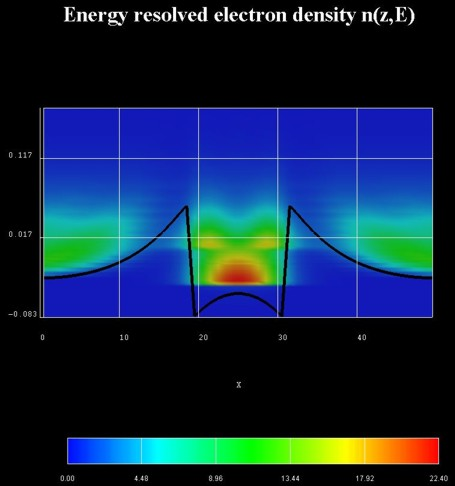Integrating n(z,E) over the energy E yields the electron density n(z) which is shown in the following figure, including the self-consistently calculated conduction band edge. The Fermi level EF is also shown.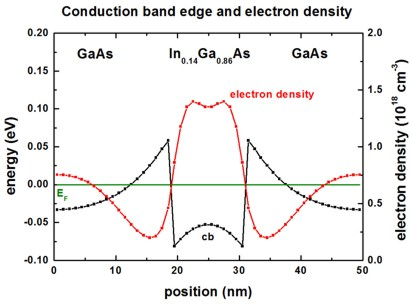## NEGF calculation including scattering - 150 mV bias

```-> 1DNEGF_InGaAs_QW_scattering_bias.in  ```

Acoustic (both, elastic and inelastic) and polar-optical phonon scattering, electron-electron scattering in Hartree approximation, see Fig. 1 (b) and 2 (a) in` [Kubis_HCIS14_2005]`.

• VSD = 0.150 eV (source-drain), see Fig. 2 (a) in``` [Kubis_HCIS14_2005]```.

### Results

The following two figures show the energy resolved electron density and the energy resolved local density of states for the QW under an applied bias of 0.150 V.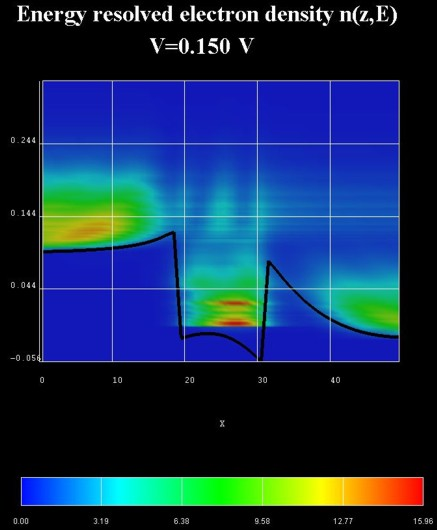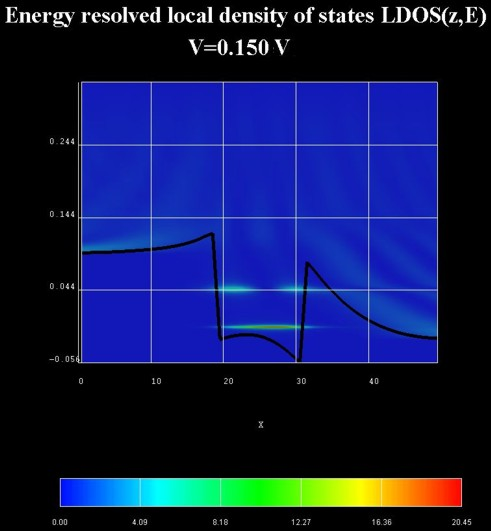The following figure shows the energy resolved current density j(z,E) in units of` [Ampere/(cm^2 eV)]`.
The relevant data is contained in these files:``` NEGF/EnergyResolvedCurrent_avs.v / *.dat / *.coord / *.fld```.The following figure shows the self-consistently calculated conduction band profile and the electron density n(z).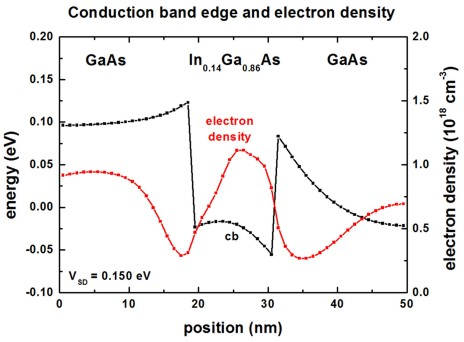### Boundary conditions for the Poisson equation

``` \$global-parameters-NEGF   ...   !----------------------------------------------------------------------   ! for Poisson equation: flat band boundary condition   ! ==> Neumann boundary condition: d phi / d z = 0   !----------------------------------------------------------------------   given_slope   = yes       ! ```The slope of the poisson potential at the contacts is fixed: flat band```   poisson_slope = 0d0       ! [V/Angstrom]   chemical_potential = 0d0  ! [eV] ``` chemical potential (Fermi level) at the right contact```   zero_drift         = yes  ! yes = ``` equilibrium contacts

### Grid points - Resolution

``` \$global-parameters-NEGF   grid_points_in_z  =  50   ! ```number of grid points in real space along z direction.
```                            ! ```It must hold:``` nextnano3 grid points - 1 = grid_points_in_z   grid_points_in_E  =  80   ! E = ```total energy```   grid_points_in_Ez = 120   ! k|| ``` resolution,``` Ez = E - hbar2 * k||2 / [2m(1)], 1 = ```1st grid point

### Applied bias (a voltage sweep is optional)

``` \$poisson-boundary-conditions   !----------------------------------------------------------------   ! ```Note: For NEGF, the voltage sweep applies to the left contact.```   !----------------------------------------------------------------   poisson-cluster-number  = 1        ! ```Source (left contact)```   region-cluster-number   = 1        ! ```an integer number which refers to an existent region-cluster```  !applied-voltage         = 0.0d0    ! 0     V           ! [V] ```apply voltage to poisson-cluster```   applied-voltage         = 0.150d0  ! 0.155 V = 150 mV  ! [V] ```apply voltage to poisson-cluster```   boundary-condition-type = ohmic   contact-control         = voltage   poisson-cluster-number  = 2        ! ```Drain (right contact)```   region-cluster-number   = 4        ! ```an integer number which refers to an existent region-cluster```   applied-voltage         = 0.0d0    ! 0 V               ! [V] ```apply voltage to poisson-cluster```   boundary-condition-type = ohmic   contact-control         = voltage```

This keyword is necessary in order to loop over different voltages.```  \$voltage-sweep   sweep-number           = 1         !   sweep-active           = no        ! 'yes'/'no'   poisson-cluster-number = 1         ! Note: For NEGF, the voltage sweep applies to the left contact.   step-size              = 0.010d0   ! [V]    0.010 V = 10 mV   number-of-steps        = 5 !  ```

Chemical potentials (often called Fermi levels) at the contacts:``` ==> ```right contact = chemical potential```              ! applied-voltage = 0.0d0 ==> ```left   contact = right contact + e * applied voltage```   ! applied-voltage = 0.150d0 (preliminary, has to be generalized!)```

• Please help us to improve our tutorial. Send comments to``` support [at] nextnano.com```.